Home | Gadget | Fuzzy | Lecturer  | Power  | Transceiver Find more type here... DECIMAL to BCD ENCODER     Encoders are the opposite of decoders. They are used to generate a coded output from a single active numeric input. To illustrate this in a simple manner, let’s take a look at the simple decimal-to-BCD encoder circuit shown below. In this circuit, normally all lines are held high by the pull-up resistors connected to +5 V. To generate a BCD output that is equivalent to a single selected decimal input, the switch corresponding to that decimal is closed. (The switch acts as an active-low input.) The truth table in Fig. 12.39 explains the rest. Figure 12.40 shows a 74LS147 decimal-to-BCD (10-line-to-4-line) priority encoder IC. The 74LS147 provides the same basic function as the circuit shown in Fig. 12.39, but it has active-low outputs. This means that instead of getting an LLHH output when “3” is selected, as in the previous encoder, you get HHLL. The two outputs represent the same thing (“3”); one is expressed in positive true logic, and the other (the 74LS147) is expressed in negative true logic. If you do not like negative true logic, you can slap inverters on the outputs of the 74LS147 to get positive true logic. The choice to use positive or negative true logic really depends on what you are planning to drive. For example, negative true logic is useful when the device that you wish to drive uses active-low inputs.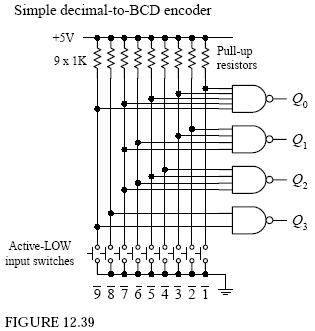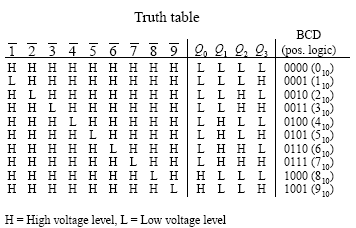74LS147 decimal-to-4-bit BCD Priority Encoder IC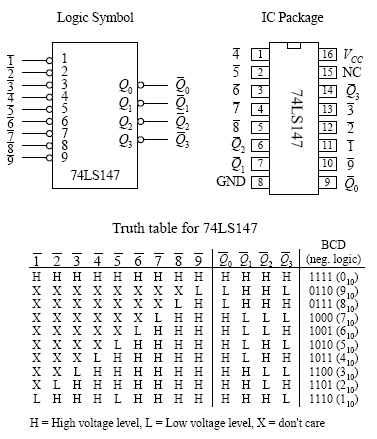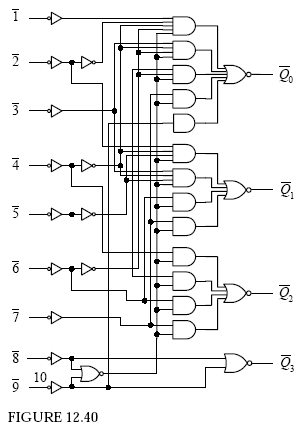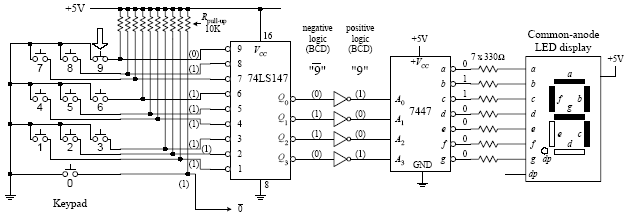Another important difference between the two encoders is the term priority that is used with the 74LS147 and not used with the encoder in Fig. 12.39. The term priority is applied to the 74LS147 because this encoder is designed so that if two or more inputs are selected at the same time, it will only select the larger-order digit. For example, if 3, 5, and 8 are selected at the same time, only the 8 (negative true BCD LHHH or 0111) will be output. The truth table in Fig. 12.40 demonstrates this look at the “don’t care” or “X” entries. With the non priority encoder, if two or more inputs are applied at the same time, the output will be unpredictable. The circuit shown in Fig. 12.41 provides a simple illustration of how an encoder and a decoder can be used together to drive an LED display via a 0-to-9 keypad. The 74LS147 encodes a keypad’s input into BCD (negative logic). A set of inverters then converts the negative true BCD into positive true BCD. The transformed BCD is then fed into a 7447 seven-segment LED display decoder/driver IC. Figure 12.42 shows a 74148 octal-to-binary priory encoder IC. It is used to transform a specified single octal input into a binary 3-bit output code. As with the 74LS147, the 74148 comes with a priority feature, meaning, again, that if two or more inputs are selected at the same time, only the higher order number is selected. complement and ten’s complement. The active-high BCD code is applied to inputs A through E. The G input is an active-low enable input. This material comes from any source reference and the copyright is own the author him self not this sites Copyright 2009 (c) technosains.com  All Rights Reserved